Study Guide

# Stoichiometry Questions

## Stoichiometry Questions

1. Copper has two stable isotopes 6329Cu (atomic mass = 62.93 amu; 69.09% natural abundance) and 6529Cu (atomic mass = 64.9278 amu; 30.91 % natural abundance). Calculate the average atomic mass of copper.

2. How many atoms of sulfur are in 25.1 g of S?

3. Calculate the molecular mass (in amu) of caffeine (C8H10N4O2).

4. How many hydrogen atoms are present in 43.8 g of urea [(NH2)2CO]?

5. Phosphoric acid is H3PO4. Calculate the percent composition by mass of H, P, and O in this compound.

6. Balance the following equation with smallest whole number coefficients:

Al + O2 → Al2O3

7. Consider the reaction: C6H12O6 + 6 O2 → 6 CO2+ 6 H2O. If 968 g of C6H12O6 is consumed, what is the mass of CO2 produced?

8. Consider the following reaction: 2 Li + 2 H2O → 2 LiOH + H2. How many grams of Li are needed to produce 7.79 g of H2?

9. Consider the following equation: 2 NH3 + CO2 → (NH2)2CO + H2O. If 849.2 g of NH3 is treated with 1223 g of CO2 how much (NH2)2CO is formed?

10. Consider the following reaction: C6H12O6+ 6 O2 → 6 CO2 + 6 H2O. How many grams of oxygen are needed to make 550 grams of CO2?

1. Copper has two stable isotopes 6329Cu (atomic mass = 62.93 amu; 69.09% natural abundance) and 6529Cu (atomic mass = 64.9278 amu; 30.91 % natural abundance). Calculate the average atomic mass of copper.

Each isotope contributes to the average atomic mass based on its relative abundance. Multiplying the mass of an isotope by its fractional abundance will give the contribution to the average atomic mass of that isotope.

(0.6909)(62.93 amu) + (0.3091)(64.9278 amu) = 63.55 amu

2. How many atoms of sulfur are in 25.1 g of S?

We need two conversions: first from grams to moles and then from moles to number of particles (atoms).

The conversion factor is: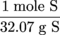1 mole = 6.023 × 1023 particles (atoms)

To solve we need to:

grams of S → moles of S → number of S atoms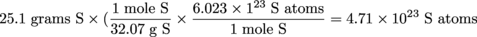3. Calculate the molecular mass (in amu) of caffeine (C8H10N4O2).

To calculate molecular mass, we need to sum all the atomic masses in the molecule. For each element, we multiply the atomic mass of the element by the number of atoms of that element in the molecule. We can find the atomic masses on the periodic table.

There are eight C atoms, ten H atoms, four N atoms, and two O atoms in caffeine, so the molecular mass is given by:

8(12.01 amu) + 10(1.008 amu) + 4(14.01 amu) + 2(16.00 amu) = 194.20 amu

4. How many hydrogen atoms are present in 43.8 g of urea [(NH2)2CO]?

We first need to calculate the molar mass of urea: 60.06 g

grams of urea → moles of urea → moles of H → atoms of H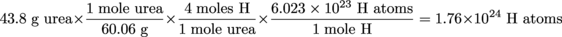5. Phosphoric acid is H3PO4. Calculate the percent composition by mass of H, P, and O in this compound.

Assume that you have 1 mole of H3PO4. The percent by mass of each element is given by the combined molar mass of the atoms of the element in 1 mole of H3PO4 divided by the molar mass of H3PO4, then multiplied by 100 percent.

The molar mass of H3PO4 is 97.99 g. That means the percent by mass of each of the elements is:6. Balance the following equation with smallest whole number coefficients:

Al + O2 → Al2O3

 Element Before After Al 1 2 O 2 3

Al +3 O2 → 2 Al2O3

 Element Before After Al 1 4 O 6 6

4 Al +3 O2 → 2 Al2O3

 Element Before After Al 4 4 O 6 6

7. Consider the reaction: C6H12O6 + 6 O2 → 6 CO2+ 6 H2O. If 968 g of C6H12O6 is consumed, what is the mass of COproduced?

Check to make sure the equation is balanced: done.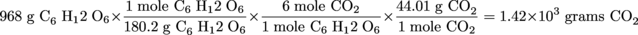8. Consider the following reaction: 2 Li + 2 H2O → 2 LiOH + H2. How many grams of Li are needed to produce 7.79 g of H2?

grams of H2→ moles of H2 → moles of Li → grams of Li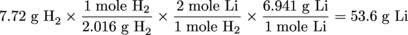9. Consider the following equation: 2 NH3 + CO2 → (NH2)2CO + H2O. If 849.2 g of NH3 is treated with 1223 g of CO2 how much (NH2)2CO is formed?

One of the reagents is the limiting reagent, but we don't know which one. We need to do two calculations. The calculation that produces the smallest amount of product is the correct answer.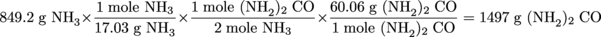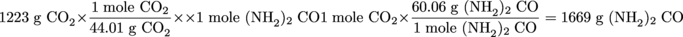10. Consider the following reaction: C6H12O6+ 6 O2 → 6 CO2 + 6 H2O. How many grams of oxygen are needed to make 550 grams of CO2?